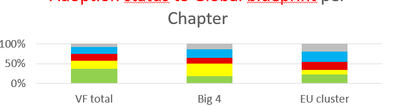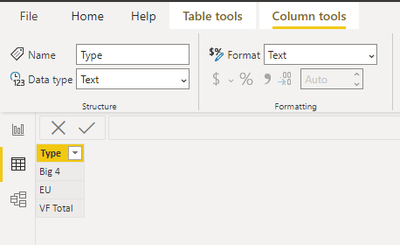cancel
Showing results for
Did you mean:

Fabric is Generally Available. Browse Fabric Presentations. Work towards your Fabric certification with the Cloud Skills Challenge.Anonymous
Not applicable

## Finding the total of two regions in same axis

Hello Team,

My question is, I have a Table stated below. Local market serves as unique coulmn, cluster is the regions comes under vodafone.

By the data, i have i can visually reprent it as the below two charts. one chart shows the two regions count and after drill trought i get the totals of both regions. Is there a way to show without drilldown, the count also in same x axis?.I want to show like this.......Regards,

1 ACCEPTED SOLUTIONCommunity Support

Hi @Anonymous ,

You can do this by customizing an axis.

1.Create a table as follows.(If the amount of data is small, you can enter data, and if the amount of data is large, you can create a calculated table.)2.Create a measure using SWITCH function or IF function.

``````Measure =
VAR _COUNT=CALCULATE(COUNT('Table'[Local Market]),FILTER(ALLSELECTED('Table'),[Cluster]=MAX('Table (2)'[Type])))
RETURN
SWITCH(SELECTEDVALUE('Table (2)'[Type]),"Big 4",_COUNT,"EU",_COUNT,"VF Total",CALCULATE(COUNT('Table'[Local Market]),ALLSELECTED('Table')))````````````Measure 2 =
VAR _COUNT=CALCULATE(COUNT('Table'[Local Market]),FILTER(ALLSELECTED('Table'),[Cluster]=MAX('Table (2)'[Type])))
RETURN
IF(SELECTEDVALUE('Table (2)'[Type])="VF Total",CALCULATE(COUNT('Table'[Local Market]),ALLSELECTED('Table')),_COUNT)
``````Best Regards,

Stephen Tao

If this post helps, then please consider Accept it as the solution to help the other members find it more quickly.

3 REPLIES 3Community Support

Hi @Anonymous ,

You can do this by customizing an axis.

1.Create a table as follows.(If the amount of data is small, you can enter data, and if the amount of data is large, you can create a calculated table.)2.Create a measure using SWITCH function or IF function.

``````Measure =
VAR _COUNT=CALCULATE(COUNT('Table'[Local Market]),FILTER(ALLSELECTED('Table'),[Cluster]=MAX('Table (2)'[Type])))
RETURN
SWITCH(SELECTEDVALUE('Table (2)'[Type]),"Big 4",_COUNT,"EU",_COUNT,"VF Total",CALCULATE(COUNT('Table'[Local Market]),ALLSELECTED('Table')))````````````Measure 2 =
VAR _COUNT=CALCULATE(COUNT('Table'[Local Market]),FILTER(ALLSELECTED('Table'),[Cluster]=MAX('Table (2)'[Type])))
RETURN
IF(SELECTEDVALUE('Table (2)'[Type])="VF Total",CALCULATE(COUNT('Table'[Local Market]),ALLSELECTED('Table')),_COUNT)
``````Best Regards,

Stephen Tao

If this post helps, then please consider Accept it as the solution to help the other members find it more quickly.Super User

@Anonymous , in the last visual from where you got the other two values (other than Big 4)Anonymous
Not applicable

Heelo,

That is ppt, I need to create a visual like that.

I could not bale to creat ewhile visualising in power biAnnouncements#### Power BI Monthly Update - November 2023

Check out the November 2023 Power BI update to learn about new features.#### The largest Power BI and Fabric virtual conference

130+ sessions, 130+ speakers, Product managers, MVPs, and experts. All about Power BI and Fabric. Attend online or watch the recordings.Top Solution Authors
Top Kudoed Authors
Users online (6,410)